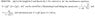# Freefall boulder problem

• Billy_Bob
Just remember to always carefully read and interpret the given information in a problem. In summary, the conversation discusses a physics problem involving a boulder falling from a cliff. The hiker notes that it takes 1.3 seconds for the boulder to fall to the last third of the way to the ground. The question asks for the height of the cliff, and the conversation includes a solution that uses the equations h = gt^2/2 and 2h/3 = gt^2/2. The expert summarizer explains that the mistake made in the original attempt is assuming that the time it takes for the boulder to fall 2h/3 is the same as the time it takes to fall h/3. Finally, the

#### Billy_Bob

This isn't really homework, but I'll be taking intro Physics in fall and am trying to get a grasp on the material ahead of time so I can do well.

## Homework Statement

An alert hiker sees a boulder fall from the top of a distant cliff and notes that it takes 1.3s for the boulder to fall to the last third of the way to the ground. You may ignore air resistance.

A. What is the heigh of the claff in meters?

## Homework Equations

What is wrong with how I did it described below, and why does the answer in the solution book say (2/3)h=(1/2)(g)(T - DeltaT)^2 with h being total height of the cliff, DeltaT being 1.3s, and T being the total time to hit the ground? Shouldn't it be (2/3)h=(1/2)(g)(DeltaT)^2

## The Attempt at a Solution

I thought: Amount fallen = (2/3)(Total height) = (1/2)(g)(t)^2
(Total height) = (3/2)(1/2)(g)(t)^2 = (3/2)(1/2)(9.8)(1.3)^2 = 12.4m

The solution they provide is the attached image.

#### Attachments

•2.98-Solution.jpg
15.2 KB · Views: 1,884
You're not thinking about this quite right. Setting it up as you've shown, you've assumed that the time it takes for the boulder to fall 2h/3 is the same as the time it takes to fall h/3.

We know that,

h = g(t^2)/2, where h represents the total height and t represents the total time. Since, it takes some known time, delta(t) to fall the last third (h/3), we know that the time it took to fall the first 2/3 of the distance was t - delta(t).

Therefore, 2h/3 = g(t - delta(t))^2/2.

"You're not thinking about this quite right. Setting it up as you've shown, you've assumed that the time it takes for the boulder to fall 2h/3 is the same as the time it takes to fall h/3."

Why have I assumed that? "(1/2)(g)(t)^2" is a unit of distance. Didn't I just find the distance to 2/3 of the way down the cliff and multiply that by 3/2 to find the distance the whole way down the cliff?

I understand they way you explain that last equation, but that's not how they explain it. Everything is the same except they define DeltaT to be the time it takes to fall from the top of the cliff to 2/3 of the way down, which is 1.3s. You define it to be the time to fall from 2/3 of the way down to the bottom.

No, that is not what you have done at all. The 1.3 s represents the time the object takes to fall the last third of the way down, after it has already been accelerating for a distance of 2h/3. Hence, you did not "just find the distance to 2/3 of the way down the cliff..." but rather the distance the boulder would fall if it fell from rest for 1.3 s.

Also, I defined delta(t) = 1.3 s exactly as you stated in your initial question, especially since the hiker notes that the it took 1.3 s for the boulder to fall the last third of the height. Since your attachment is still pending approval I cannot see how they have done it.

^Ahh, I was misunerstanding the problem. I thought it was saying 1.3 sec was the time to fall from the top of the cliff to the top of the bottom third of the cliff (hence going 2/3 of the way down).

Thanks

No problem.